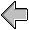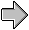# Complete Program from Figure 13

```(define Basic-Shapes
(unit (import)
(export Shape Rectangle Circle Translated)

(define Shape (interface () draw))

(define Rectangle
(class* object% (Shape) (width height)
(public
[draw (lambda (dc x y)
(send dc draw-rectangle x y width height))])
(sequence (super-init))))

(define Circle
(public
[draw (lambda (dc x y)
(send dc draw-ellipse
(sequence (super-init))))

(define Translated
(class* object% (Shape) (orig-shape dx dy)
(public
[draw (lambda (dc x y)
(send orig-shape draw
dc (+ x dx) (+ y dy)))])
(sequence (super-init))))))

(define Union-Shape
(unit (import Shape)
(export Union)

(define Union
(class* object% (Shape) (left right)
(public
[draw (lambda (dc x y)
(send left draw dc x y)
(send right draw dc x y))])
(sequence (super-init))))))

(define Basic+Union-Shapes
(compound-unit
(import)
[US (Union-Shape (S Shape))])
(export(S Shape)
(S Rectangle)
(S Circle)
(S Translated)
(US Union))))

(define BB-Shapes
(unit (import Shape Rectangle Circle Translated Union)
(export BB-Shape BB-Rectangle BB-Circle
BB-Translated BB-Union
make-BB BB-left BB-top BB-right BB-bottom)

(define BB-Shape (interface (Shape) bounding-box))

(define-struct BB (left top right bottom))

(define BB-Rectangle
(class* Rectangle (BB-Shape) (width height)
(public
[bounding-box
(lambda () (make-BB 0 0 width height))])
(sequence (super-init width height))))

(define BB-Circle
(class* Circle (BB-Shape) (r)
(public
[bounding-box
(lambda () (make-BB (- r) (- r) r r))])
(sequence (super-init r))))

(define BB-Translated
(class* Translated (BB-Shape) (shape dx dy)
(public
[bounding-box
(lambda ()
(let ([pre-bb (send shape bounding-box)])
(make-BB (+ (BB-left pre-bb) dx)
(+ (BB-top pre-bb) dy)
(+ (BB-right pre-bb) dx)
(+ (BB-bottom pre-bb) dy))))])
(sequence (super-init shape dx dy))))

(define BB-Union
(class* Union (BB-Shape) (left right)
(public
[bounding-box
(lambda ()
(let ([left-bb (send left bounding-box)]
[right-bb (send right bounding-box)])
(make-BB (min (BB-left left-bb) (BB-left right-bb))
(min (BB-top left-bb) (BB-top right-bb))
(max (BB-right left-bb) (BB-right right-bb))
(max (BB-bottom left-bb) (BB-bottom right-bb)))))])
(sequence (super-init left right))))))

(define Basic+Union+BB-Shapes
(compound-unit
(import)
[BS (BB-Shapes (S Shape)
(S Rectangle)
(S Circle)
(S Translated)
(S Union))])
(export (S Shape)
(BS BB-Shape)

;rename BS's BB-Rectangle to Rectangle, etc.:
(BS (BB-Rectangle Rectangle))
(BS (BB-Circle Circle))
(BS (BB-Translated Translated))
(BS (BB-Union Union))

(BS make-BB)
(BS BB-left)
(BS BB-top)
(BS BB-right)
(BS BB-bottom))))

(define Picture
(unit (import Rectangle Circle Translated display-shape)
(export shape1 shape2)

(define shape1 (make-object Rectangle 40 30))
(define shape2 (make-object Translated
(make-object Circle 20)
30 30))

(display-shape shape1)
(display-shape shape2)))

(define Union-Picture
(unit (import Rectangle Circle Translated Union
shape1 shape2
display-shape)
(export)

(define shape3 (make-object Union shape1 shape2))
(display-shape shape3)))

;; the graphics library (mred@) is defaultly a signed unit.
;; see mzscheme manual for details of signed units.
(define MrEd-Toolkit (unit/sig->unit mred@))

(define BB-Gui
(unit (import BB-Shape
BB-left BB-top BB-right BB-bottom
canvas% frame%)
(export display-shape)

(define shape-canvas%
(class canvas% (parent shape)
(inherit get-dc get-client-size)
(override
[on-paint
(lambda ()
(let-values ([(win-width win-height) (get-client-size)])
(let* ([bb (send shape bounding-box)]
[size
(lambda (left1 right1 left2 right2)
(- (/ (- (- right2 left2)
(- right1 left1))
2)
left1))]
[x (size (BB-left bb) (BB-right bb) 0 win-width)]
[y (size (BB-top bb) (BB-bottom bb) 0 win-height)])
(send shape draw (get-dc) x y))))])
(sequence (super-init parent))))

(define display-shape
(lambda (a-shape)
(unless (is-a? a-shape BB-Shape)
(error 'display-shape "expected a BB-Shape, got: ~e" a-shape))
(let* ([frame (make-object frame% "Centered Shapes" #F 150 150)]
[canvas (make-object shape-canvas% frame a-shape)])
(send frame show #t))))))

(define BB-Program
(compound-unit
(import)
[M (MrEd-Toolkit)]
[BG (BB-Gui (S BB-Shape)
(S BB-left) (S BB-top) (S BB-right) (S BB-bottom)
(M canvas%) (M frame%))]
[P (Picture (S Rectangle)
(S Circle)
(S Translated)
(BG display-shape))]
[UP (Union-Picture (S Rectangle)
(S Circle)
(S Translated)
(S Union)
(P shape1)
(P shape2)
(BG display-shape))])
(export)))

(invoke-unit BB-Program)

```figurein context contents